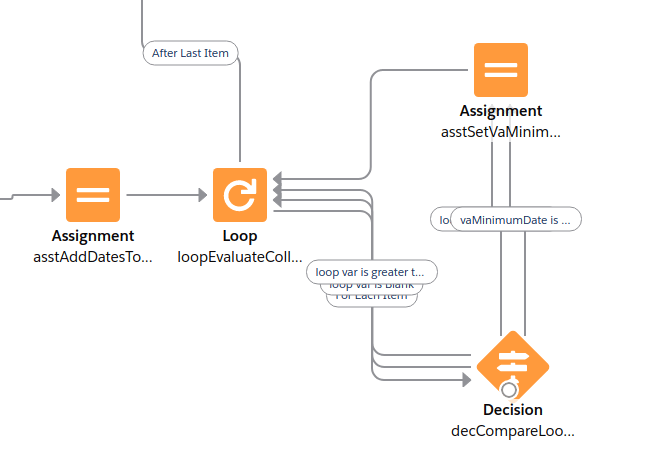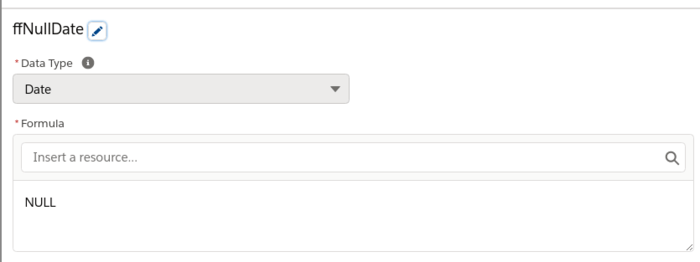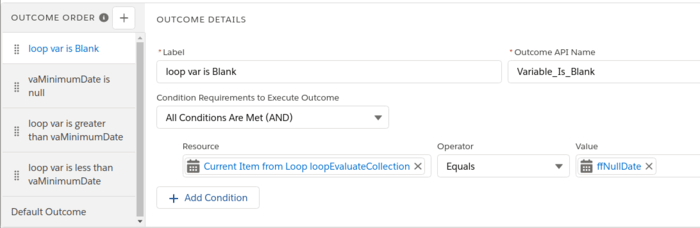# Evaluating max/min of multiple date fields in flow

I have 3 date fields on an object and i need the MIN of the 3. But the MIN formula function does not allow dates, just numbers

IF could work for up to 3, and there are some solutions out there using formulas, but seemed fragile / complex.

So, solved via flow

1. create a variable, type = date; name it vaMinimumDate

2. use an assignment element to add all the date fields from the object to a collection variable (doesnt matter if the dates are null or not)

3. loop over the collection variable

4. in the loop, use a decision to evaluate if the current loop variable is less than vaMinimumDate

ScreenshotNotes
To test if the date field is blank/null, create a formula field, type = date, value = NULL, and then in the decision you can test equals/not equals formulaField.The outcomes in the decision need to be in this order
a) is loop variable null - if so, pass on this iteration
b) is vaMinimumDate null - if so set it to current loop variable (which is not blank, bc else it would get caught by step above)
c) is loop variable > vaMinimumDate - if so, pass on this iteration
d) is loop variable <= vaMinimumDate - if so update vaMinimumDateTested a bunch and seems to be working properly. Let me know if you find otherwise!